## Problem Solving: Guess and Check## Teach students the same technique research mathematicians use! (Seriously.)

Need more tips and tricks for teaching math? You can find them in our math resources center .

## What Is It?

"Guess and Check" is a problem-solving strategy that students can use to solve mathematical problems by guessing the answer and then checking that the guess fits the conditions of the problem. For example, the following problem would be best solved using guess and check:

Of 25 rounds at the regional spelling contest, the Mighty Brains tied 3 rounds and won 2 more than they lost. How many rounds did the Mighty Brains win?

## Why Is It Important?

All research mathematicians use guess and check, and it is one of the most powerful methods of solving differential equations, which are equations involving an unknown function and its derivatives. A mathematician's guess is called a "conjecture" and looking back to check the answer and prove that it is valid, is called a "proof." The main difference between problem solving in the classroom and mathematical research is that in school, there is usually a known solution to the problem. In research the solution is often unknown, so checking solutions is a critical part of the process.

## How Can You Make It Happen?

Introduce a problem to students that will require them to make and then check their guess to solve the problem. For example, the problem:

Ben knows 100 baseball players by name. Ten are Red Sox. The rest are Blue Jays and Diamondbacks. He knows the names of twice as many Diamondbacks as Blue Jays. How many Blue Jays does he know by name?

When students use the strategy of guess and check, they should keep a record of what they have done. It might be helpful to have them use a chart or table.

## Understand the Problem

Demonstrate that the first step is understanding the problem. This involves finding the key pieces of information needed to find the answer. This may require reading the problem several times, and/or students putting the problem into their own words.

For example, "I know there are twice as many Diamondbacks as Blue Jays. There are 10 Red Sox. The number of Blue Jays and Diamondbacks should equal 90."

Choose a Strategy

Use the "Guess and Check" strategy. Guess and check is often one of the first strategies that students learn when solving problems. This is a flexible strategy that is often used as a starting point when solving a problem, and can be used as a safety net, when no other strategy is immediately obvious.

Featured high school resources.## Related Resources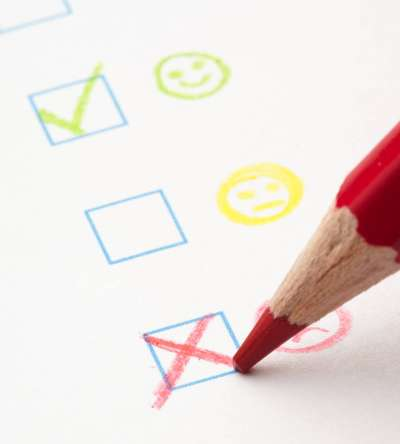## TeacherVision Editorial Staff

The TeacherVision editorial team is comprised of teachers, experts, and content professionals dedicated to bringing you the most accurate and relevant information in the teaching space.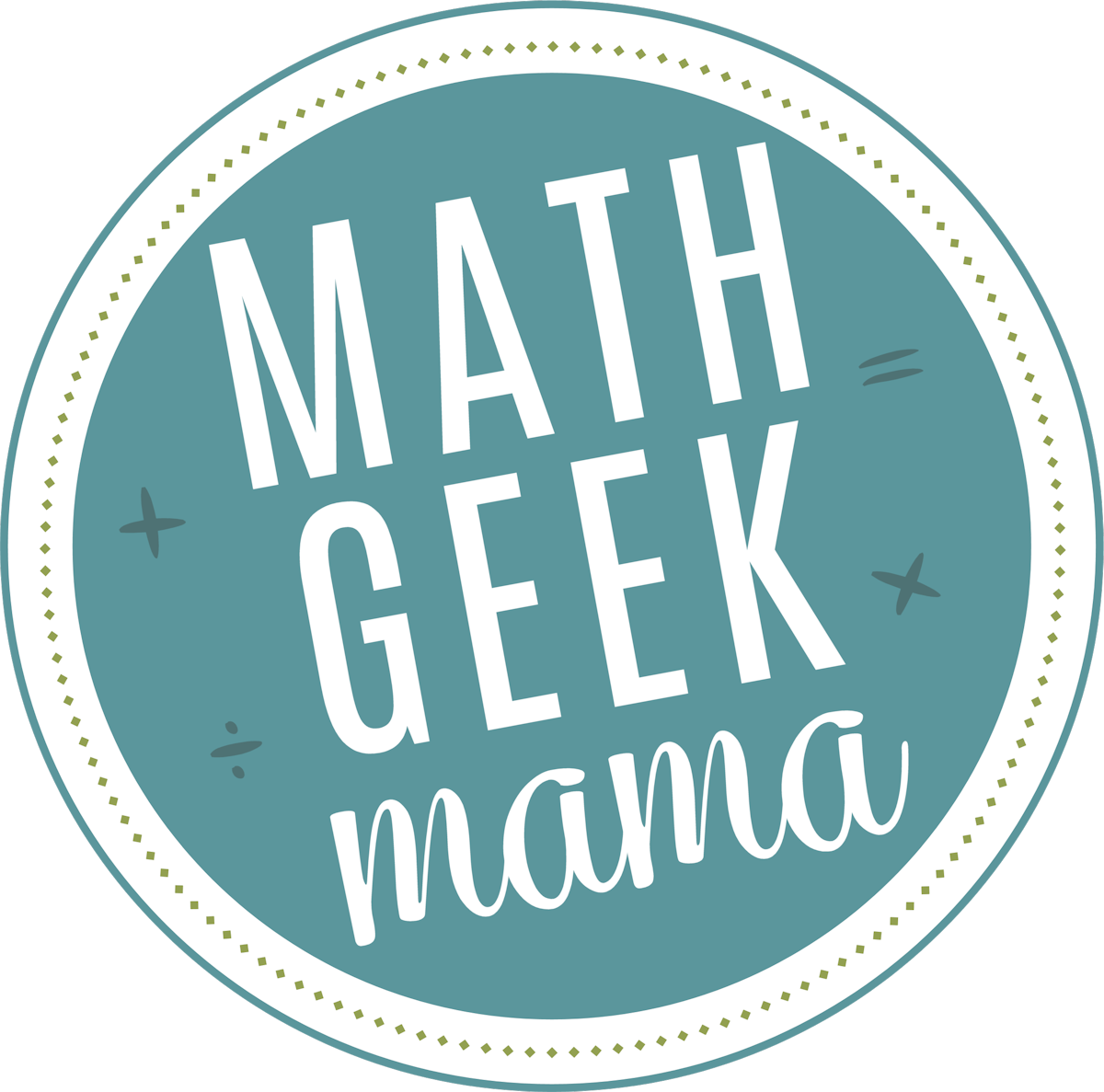## Fun teaching resources & tips to help you teach math with confidence## Solving Problems Using Guess and Check

Welcome to the last week in my series on problem solving strategies ! There are so many different ways to approach math word problems, but it’s important that we share these various methods with kids so that they can be equipped to tackle them! This week I’m explaining a strategy that doesn’t sound overly mathematical, but can be extremely useful when done properly: solving problems using guess and check ! As with the other strategies I’ve discussed, it’s important to help kids understand how to use this method so that they are not randomly pulling answers out of their head and wasting time.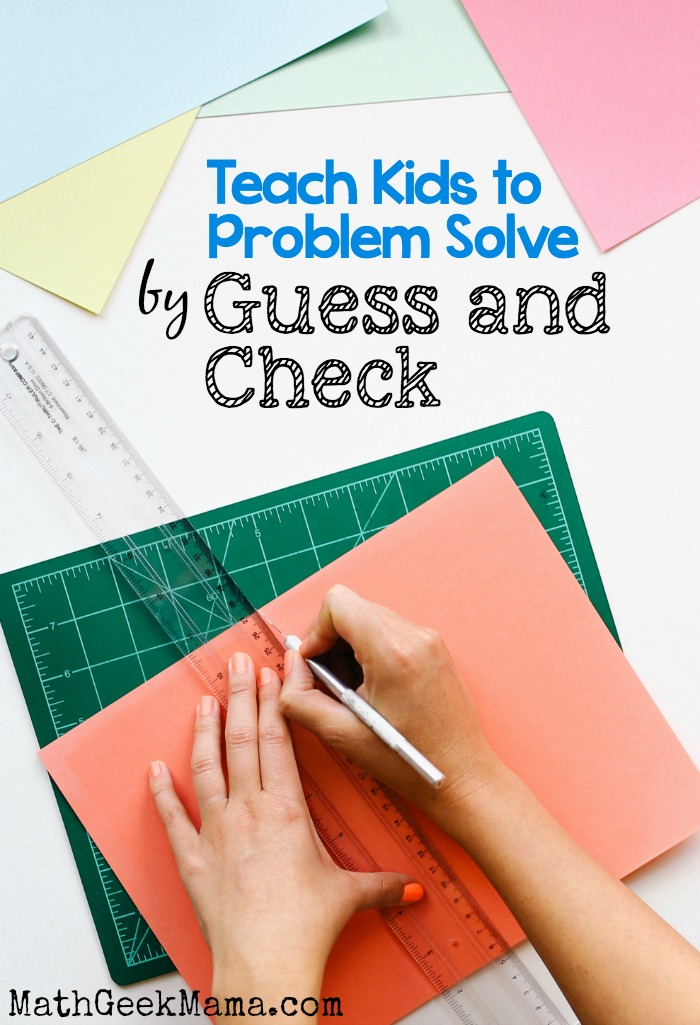–>Pssst! Do your kids need help making sense of and solving word problems? You might like this set of editable word problem solving templates ! Use these with any grade level, for any type of word problem :

## Guess & Check Math Strategy:

You may hear the name of this strategy and think, “Guess? Isn’t the whole point of math instruction to teach kids to solve problems so that they’re no longer merely guessing ??” While it is certainly true that we don’t want kids to simply guess random answers for every math problem they ever encounter, there are instances when educated guesses are important, valid and useful.

For instance, learning and understanding how to accurately estimate is an important mathematical skill. A good estimate, however, is not just a random guess. It takes effort and logic to formulate an estimate that makes sense and is (hopefully) close to the correct answer. (For fun and easy estimation practice, try this Mummy Math activity !)

Similarly, solving problems using guess and check is a process that requires logic and an understanding of the question so that it can be done in a way that is organized and time saving.

So what does guess and check mean? To be more specific, this strategy should be called, “Guess, Check and Revise.”

## The basic structure of the strategy looks like this:

• Form an educated guess
• Check your solution to see if it works and solves the problem
• If not, revise your guess based on whether it is too high or too low

This is a useful strategy when you’re given the total and you’re asked to find the kinds or number of things making up the total. Or when the question asks for the value of two or more different kinds of things.

For instance, you might be asked how many girls and how many boys are in the class, or how many cats and how many dogs a pet owner has.

When guess and check seems like an appropriate strategy for a word problem, it will be helpful and necessary to then organize the information in a table or list to keep track of the different guesses. This provides a visual of the important information, and will also help ensure that subsequent guesses are logical and not random.

## Using the Guess and Check Strategy:

To begin, students should make a guess using what they know from the problem. This first guess can be anything at all, so long as it follows the criteria given. Then, once a guess is made, students can begin to make more educated guesses based on how close they are to the correct answer.

For example, if their initial guess gives a total that is too high, they need to choose smaller numbers for their next guess. Likewise, if their guess gives a total that is too low, they need to choose larger numbers.

The most important thing for students to understand when using this method is that after their initial guess, they should work towards getting closer to the correct answer by making logical changes to their guess. They should not be choosing random numbers anymore!

## Here’s an example to consider:

In Ms. Brown’s class, there are 24 students. There are 6 more girls than boys. How many boys and girls are there?

Because we know the class total (24), and we’re asked to find more than one value (number of boys and number of girls), we can solve this using the guess and check method.

To organize the question, we can form a table with boys, girls and the total. Because we know there are 6 more girls than boys, we can guess a number for the boys, and then calculate the girls and the total from there.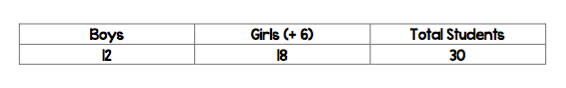With an initial guess of 12 boys, we see that there would be 18 girls, giving a total class size of 30. The total, however, should only be 24, which means our guess was too high. Knowing this, the number of boys is revised and the total recalculated .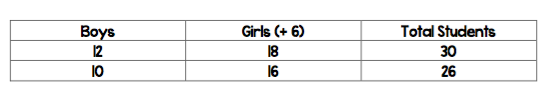Lowering the number of boys to 10 would mean there are 16 girls, which gives a class total of 26. This is still just a little bit too high, so we can once again revise the guess to 9 boys. If there are 9 boys, that would mean there are 15 girls, which gives a class total of 24.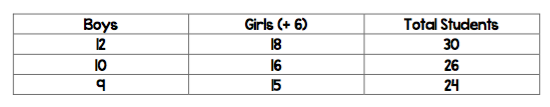Therefore, the solution is 9 boys and 15 girls.

This is a fairly simple example, and likely you will have students who can solve this problem without writing out a table and forming multiple guesses. But for students who struggle with math , this problem may seem overwhelming and complicated. By giving them a starting point and helping them learn to make more educated guesses , you can equip them to not only solve word problems, but feel more confident in tackling them.

This is also a good strategy because it helps kids see that it’s ok to make mistakes and that we shouldn’t expect to get the right answer on the first try, but rather, we should expect to make mistakes and use our mistake to learn and find the right answer.

What do you think? Do you use or teach this strategy to students? Do you find it helpful? Share in the comments!## And of course, don’t miss the rest of the problem solving strategy series:

• Problem Solve by Solving an Easier Problem
• Problem Solve by Drawing a Picture
• Problem Solve by Working Backwards
• Problem Solve by Making a List
• Problem Solve by Finding a Pattern

## Never Run Out of Fun Math Ideas

If you enjoyed this post, you will love being a part of the Math Geek Mama community! Each week I send an email with fun and engaging math ideas, free resources and special offers. Join 163,000+ readers as we help every child succeed and thrive in math! PLUS, receive my FREE ebook, 5 Math Games You Can Play TODAY , as my gift to you !

My strategy was usually more “guess and hope for the best”. Yours sounds much wiser, lol! Thanks for sharing at the Thoughtful Spot!

Haha!! Yes, I think that’s what most people think of when they hear, “Guess and Check!” Hope this was helpful, 🙂

How exactly do you do this?

Sorry, your way was amazing…but I’m still confused:(

I’m so sorry you’re confused. Can you explain what part you don’t understand so I can try and make it clearer? The object is to make a reasonable guess, and then adjust your guess based on if your answer is too high or too low (try to be logical rather than random).

Nicely explained post and a different logic for solving problems “Guess and check”, I don’t know that how much helpful it is but I’m sure that your idea will help to change the thinking of readers. Thanks for sharing a different kind of idea for problem solving.

## Similar Posts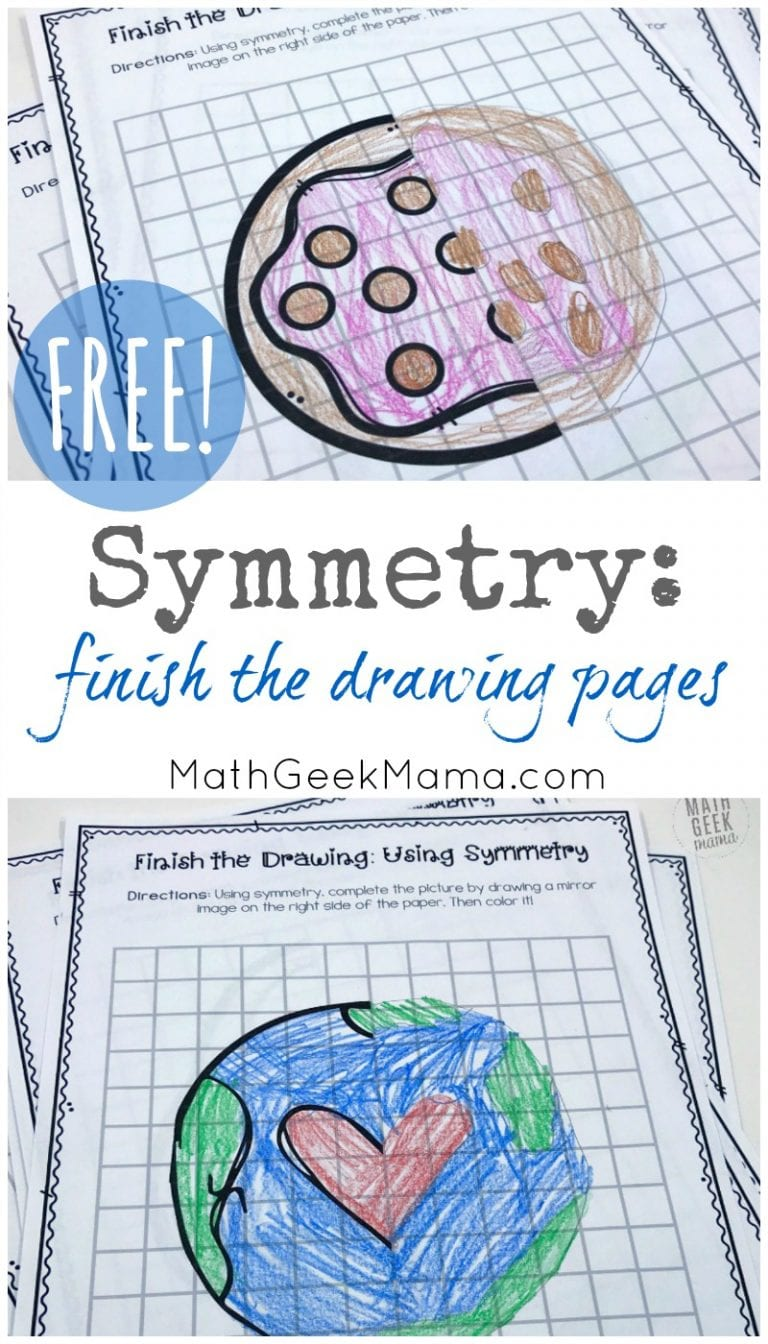## Symmetry: Finish the Picture Pages {Drawing Mirror Images}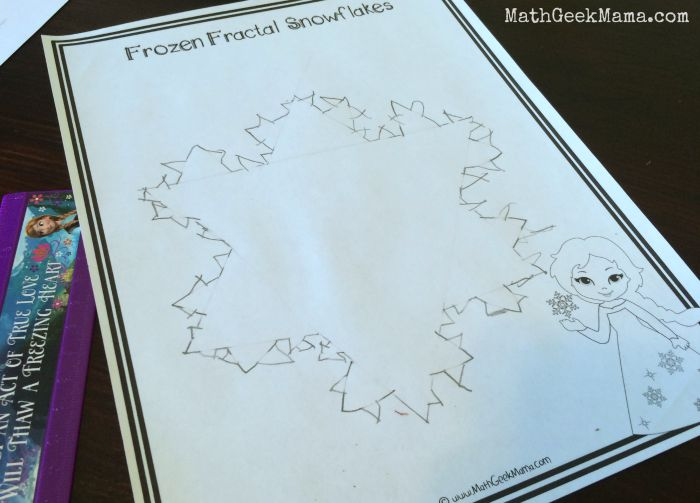## Disney Math: Frozen Fractal Snowflake {FREE Printable}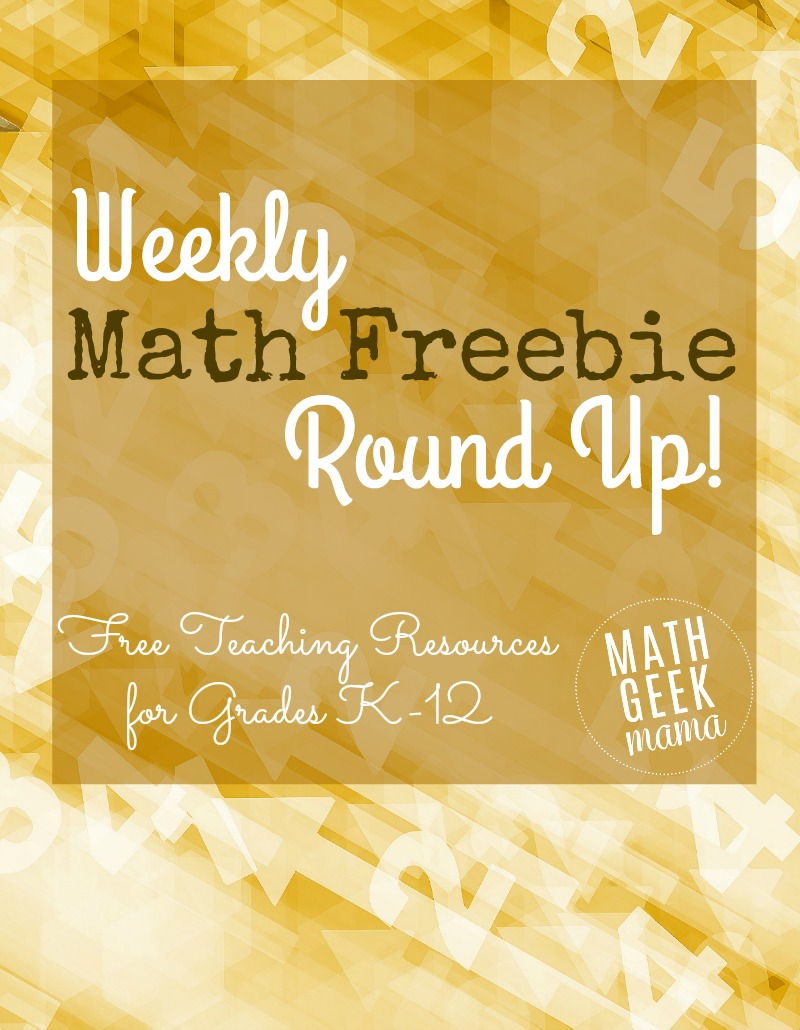## Weekly Math Freebie Round Up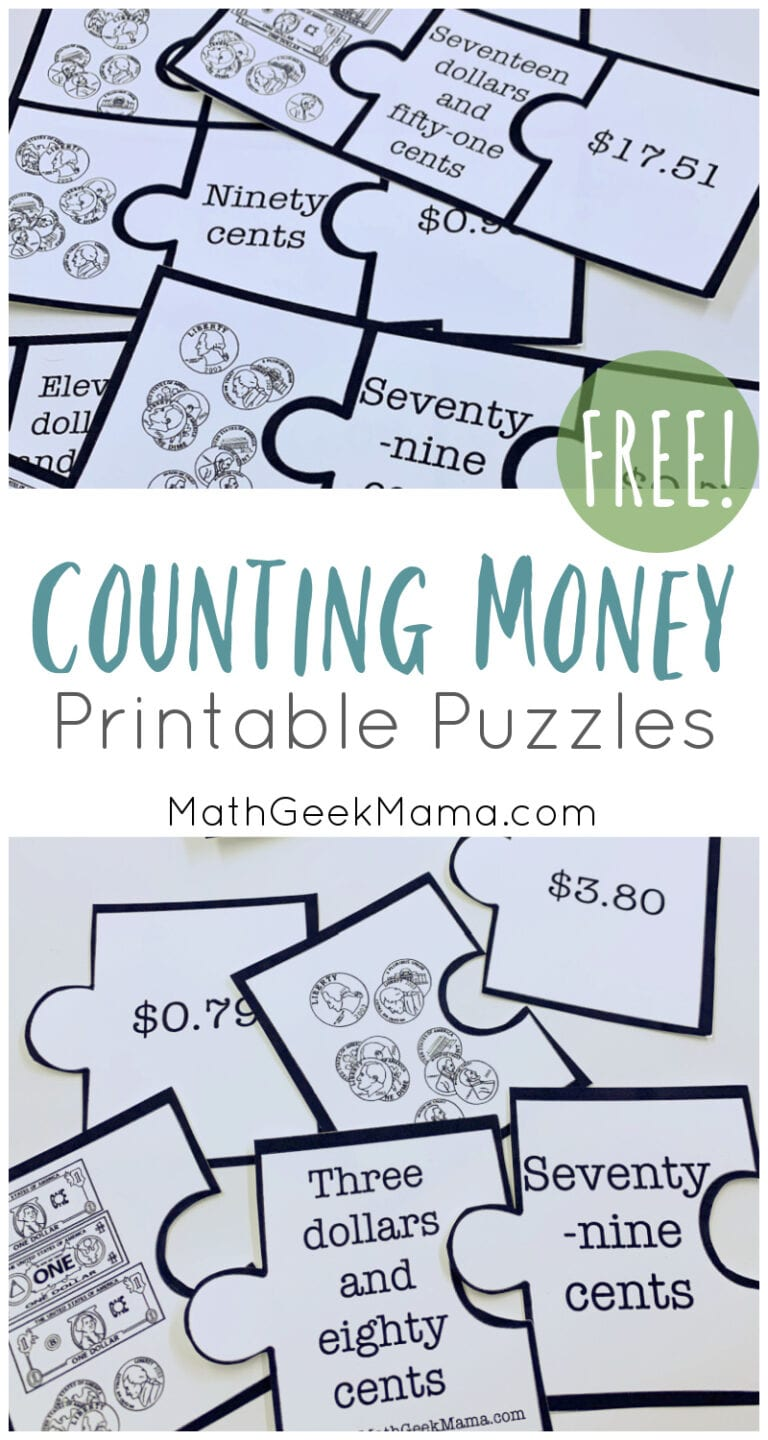## Counting Money Puzzles {FREE!}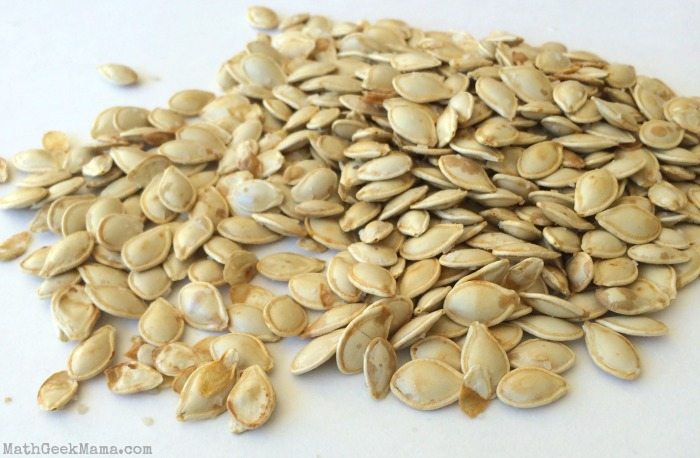## How Many Seeds in a Pumpkin? Fall Estimation Fun!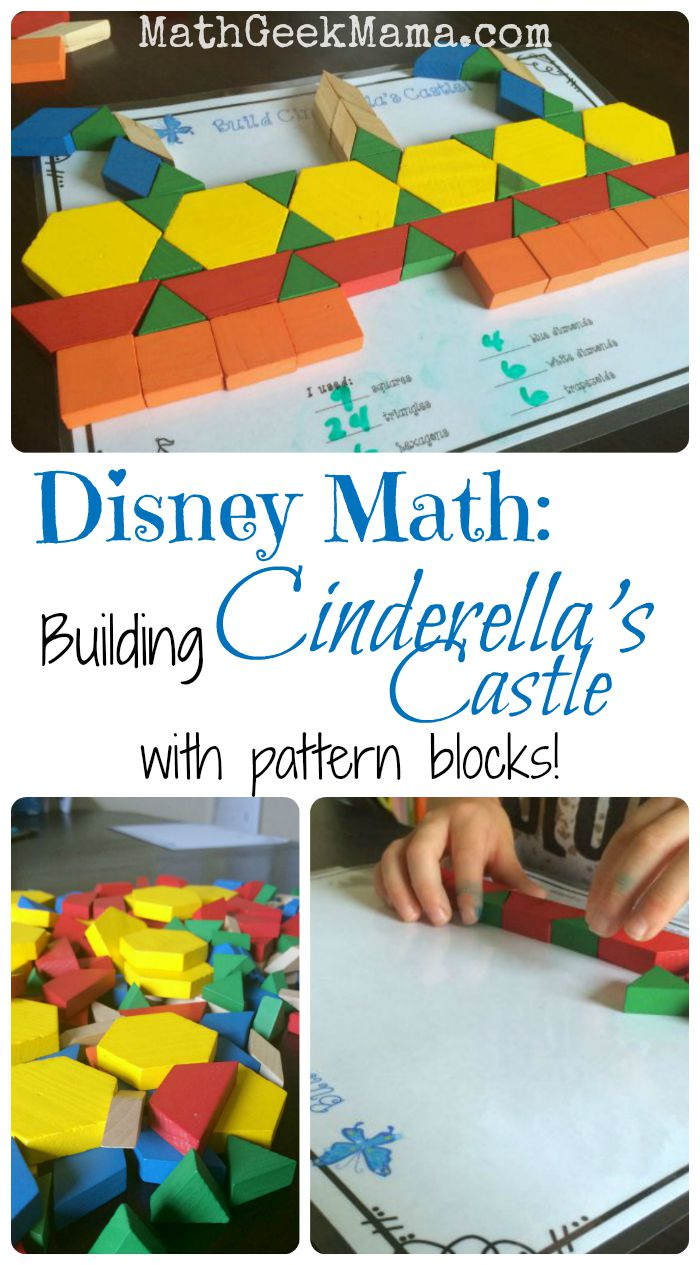## Disney Math: Using Pattern Blocks to Create Cinderella’s Castle!

Find more resources to help make math engaging, join 165k+ parents & teachers.

Who learn new tips and strategies, as well as receive engaging resources to make math fun!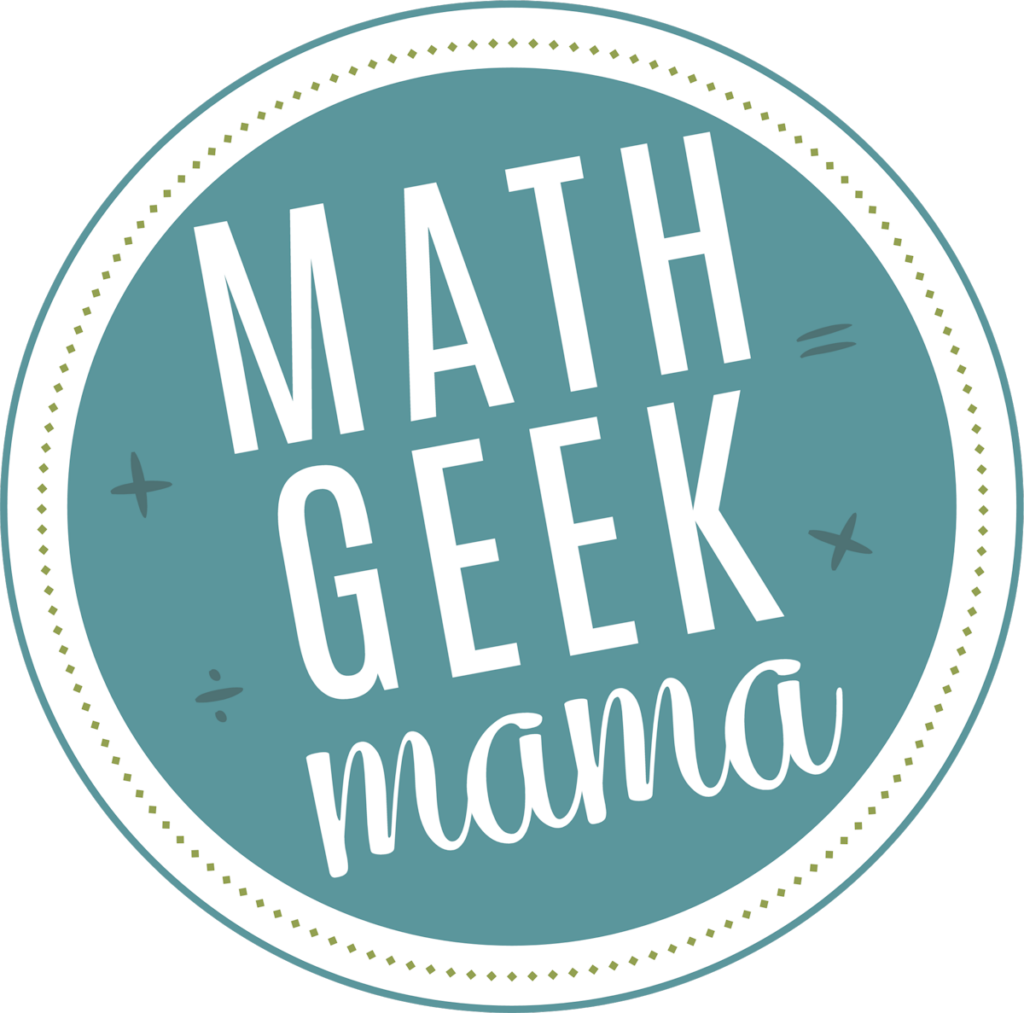## Math Time Doesn't Have to End in Tears

Join 165,000+ parents and teachers who learn new tips and strategies, as well as receive engaging resources to make math fun. Plus, receive my guide, "5 Games You Can Play Today to Make Math Fun," as my free gift to get you started!## How Do You Solve a Problem Using the Guess, Check, and Revise Method?

Some problems can be solve by guessing an answer, checking it, and then revising your guess. This tutorial goes through that process step-by-step and shows you how to solve a word problem using the guess, test, revise method!

• word problem

## Background Tutorials

Operations with whole numbers.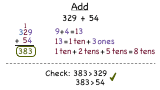## How Do You Add Whole Numbers?

To add numbers, you can line up the numbers vertically and then add the matching places together. This tutorial shows you how to add numbers vertically!

## A Problem-Solving Plan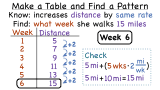## How Do You Solve a Problem by Making a Table and Finding a Pattern?

Making a table can be a very helpful way to find a pattern in numbers and solve a problem. This tutorial shows you how to take the information from and word problem to create a table and use it to find the answer!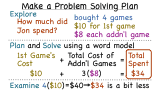## How Do You Make a Problem Solving Plan?

Planning is a key part of solving math problems. Follow along with this tutorial to see the steps involved to make a problem solving plan!

## Further Exploration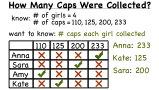## How Do You Solve a Problem Using Logical Reasoning?

Using logic is a strong approach to solving math problems! This tutorial goes through an example of using logical reasoning to find the answer to a word problem.

The home of mathematics education in New Zealand.

• Teaching material
• Problem solving activities
• Problem Solving Information

## Problem Solving Strategies

What are problem solving strategies.

Strategies are things that Pólya would have us choose in his second stage of problem solving and use in his third stage ( What is Problem Solving? ). In actual fact he called them heuristics . They are a collection of general approaches that might work for a number of problems.

There are a number of common strategies that students of primary age can use to help them solve problems. We discuss below several that will be of value for problems on this website and in books on problem solving.

Common Problem Solving Strategies

• Guess (includes guess and check, guess and improve)
• Act It Out (act it out and use equipment)
• Draw (this includes drawing pictures and diagrams)
• Make a List (includes making a table)
• Think (includes using skills you know already)

We have provided a copymaster for these strategies so that you can make posters and display them in your classroom. It consists of a page per strategy with space provided to insert the name of any problem that you come across that uses that particular strategy (Act it out, Draw, Guess, Make a List). This kind of poster provides good revision for students.

## An In-Depth Look At Strategies

We now look at each of the following strategies and discuss them in some depth. You will see that each strategy we have in our list includes two or more subcategories.

• Guess and check is one of the simplest strategies. Anyone can guess an answer. If they can also check that the guess fits the conditions of the problem, then they have mastered guess and check. This is a strategy that would certainly work on the Farmyard problem described below but it could take a lot of time and a lot of computation. Because it is so simple, you may have difficulty weaning some students away from guess and check. As problems get more difficult, other strategies become more important and more effective. However, sometimes when students are completely stuck, guessing and checking will provide a useful way to start to explore a problem. Hopefully that exploration will lead to a more efficient strategy and then to a solution.
• Guess and improve is slightly more sophisticated than guess and check. The idea is that you use your first incorrect guess to make an improved next guess. You can see it in action in the Farmyard problem. In relatively straightforward problems like that, it is often fairly easy to see how to improve the last guess. In some problems though, where there are more variables, it may not be clear at first which way to change the guessing.
• Young students especially, enjoy using Act it Out . Students themselves take the role of things in the problem. In the Farmyard problem, the students might take the role of the animals though it is unlikely that you would have 87 students in your class! But if there are not enough students you might be able to include a teddy or two. This is an effective strategy for demonstration purposes in front of the whole class. On the other hand, it can also be cumbersome when used by groups, especially if a largish number of students is involved.  Sometimes the students acting out the problem may get less out of the exercise than the students watching. This is because the participants are so engrossed in the mechanics of what they are doing that they don’t see the underlying mathematics.
• Use Equipment is a strategy related to Act it Out. Generally speaking, any object that can be used in some way to represent the situation the students are trying to solve, is equipment. One of the difficulties with using equipment is keeping track of the solution. The students need to be encouraged to keep track of their working as they manipulate the equipment. Some students need to be encouraged and helped to use equipment. Many students seem to prefer to draw. This may be because it gives them a better representation of the problem in hand. Since there are problems where using equipment is a better strategy than drawing, you should encourage students' use of equipment by modelling its use yourself from time to time.
• It is fairly clear that a picture has to be used in the strategy Draw a Picture . But the picture need not be too elaborate. It should only contain enough detail to help solve the problem. Hence a rough circle with two marks is quite sufficient for chickens and a blob plus four marks will do a pig. All students should be encouraged to use this strategy at some point because it helps them ‘see’ the problem and it can develop into quite a sophisticated strategy later.
• It’s hard to know where Drawing a Picture ends and Drawing a Diagram begins. You might think of a diagram as anything that you can draw which isn’t a picture. But where do you draw the line between a picture and a diagram? As you can see with the chickens and pigs, discussed above, regular picture drawing develops into drawing a diagram. Venn diagrams and tree diagrams are particular types of diagrams that we use so often they have been given names in their own right.
• There are a number of ways of using Make a Table . These range from tables of numbers to help solve problems like the Farmyard, to the sort of tables with ticks and crosses that are often used in logic problems. Tables can also be an efficient way of finding number patterns.
• When an Organised List is being used, it should be arranged in such a way that there is some natural order implicit in its construction. For example, shopping lists are generally not organised. They usually grow haphazardly as you think of each item. A little thought might make them organised. Putting all the meat together, all the vegetables together, and all the drinks together, could do this for you. Even more organisation could be forced by putting all the meat items in alphabetical order, and so on. Someone we know lists the items on her list in the order that they appear on her route through the supermarket.
• Being systematic may mean making a table or an organised list but it can also mean keeping your working in some order so that it is easy to follow when you have to go back over it. It means that you should work logically as you go along and make sure you don’t miss any steps in an argument. And it also means following an idea for a while to see where it leads, rather than jumping about all over the place chasing lots of possible ideas.
• It is very important to keep track of your work. We have seen several groups of students acting out a problem and having trouble at the end simply because they had not kept track of what they were doing. So keeping track is particularly important with Act it Out and Using Equipment. But it is important in many other situations too. Students have to know where they have been and where they are going or they will get hopelessly muddled. This begins to be more significant as the problems get more difficult and involve more and more steps.
• In many ways looking for patterns is what mathematics is all about. We want to know how things are connected and how things work and this is made easier if we can find patterns. Patterns make things easier because they tell us how a group of objects acts in the same way. Once we see a pattern we have much more control over what we are doing.
• Using symmetry helps us to reduce the difficulty level of a problem. Playing Noughts and crosses, for instance, you will have realised that there are three and not nine ways to put the first symbol down. This immediately reduces the number of possibilities for the game and makes it easier to analyse. This sort of argument comes up all the time and should be grabbed with glee when you see it.
• Finally working backwards is a standard strategy that only seems to have restricted use. However, it’s a powerful tool when it can be used. In the kind of problems we will be using in this web-site, it will be most often of value when we are looking at games. It frequently turns out to be worth looking at what happens at the end of a game and then work backward to the beginning, in order to see what moves are best.
• Then we come to use known skills .  This isn't usually listed in most lists of problem solving strategies but as we have gone through the problems in this web site, we have found it to be quite common.  The trick here is to see which skills that you know can be applied to the problem in hand. One example of this type is Fertiliser (Measurement, level 4).  In this problem, the problem solver has to know the formula for the area of a rectangle to be able to use the data of the problem.  This strategy is related to the first step of problem solving when the problem solver thinks 'have I seen a problem like this before?'  Being able to relate a word problem to some previously acquired skill is not easy but it is extremely important.

## Uses of Strategies

Different strategies have different uses. We’ll illustrate this by means of a problem.

The Farmyard Problem : In the farmyard there are some pigs and some chickens. In fact there are 87 animals and 266 legs. How many pigs are there in the farmyard?

Some strategies help you to understand a problem. Let’s kick off with one of those. Guess and check . Let’s guess that there are 80 pigs. If there are they will account for 320 legs. Clearly we’ve over-guessed the number of pigs. So maybe there are only 60 pigs. Now 60 pigs would have 240 legs. That would leave us with 16 legs to be found from the chickens. It takes 8 chickens to produce 16 legs. But 60 pigs plus 8 chickens is only 68 animals so we have landed nearly 20 animals short.

Obviously we haven’t solved the problem yet but we have now come to grips with some of the important aspects of the problem. We know that there are 87 animals and so the number of pigs plus the number of chickens must add up to 87. We also know that we have to use the fact that pigs have four legs and chickens two, and that there have to be 266 legs altogether.

Some strategies are methods of solution in themselves. For instance, take Guess and Improve . Supposed we guessed 60 pigs for a total of 240 legs. Now 60 pigs imply 27 chickens, and that gives another 54 legs. Altogether then we’d have 294 legs at this point.

Unfortunately we know that there are only 266 legs. So we’ve guessed too high. As pigs have more legs than hens, we need to reduce the guess of 60 pigs. How about reducing the number of pigs to 50? That means 37 chickens and so 200 + 74 = 274 legs.

We’re still too high. Now 40 pigs and 47 hens gives 160 + 94 = 254 legs. We’ve now got too few legs so we need to guess more pigs.

You should be able to see now how to oscillate backwards and forwards until you hit on the right number of pigs. So guess and improve is a method of solution that you can use on a number of problems.

Some strategies can give you an idea of how you might tackle a problem. Making a Table illustrates this point. We’ll put a few values in and see what happens.

From the table we can see that every time we change the number of pigs by one, we change the number of legs by two. This means that in our last guess in the table, we are five pigs away from the right answer. Then there have to be 46 pigs.

Some strategies help us to see general patterns so that we can make conjectures. Some strategies help us to see how to justify conjectures. And some strategies do other jobs. We’ll develop these ideas on the uses of strategies as this web-site grows.

What Strategies Can Be Used At What Levels

In the work we have done over the last few years, it seems that students are able to tackle and use more strategies as they continue with problem solving. They are also able to use them to a deeper level. We have observed the following strategies being used in the stated Levels.

Levels 1 and 2

• Draw a Picture
• Use Equipment
• Guess and Check

Levels 3 and 4

• Draw a Diagram
• Guess and Improve
• Make a Table
• Make an Organised List

It is important to say here that the research has not been exhaustive. Possibly younger students can effectively use other strategies. However, we feel confident that most students at a given Curriculum Level can use the strategies listed at that Level above. As problem solving becomes more common in primary schools, we would expect some of the more difficult strategies to come into use at lower Levels.

Strategies can develop in at least two ways. First students' ability to use strategies develops with experience and practice. We mentioned that above. Second, strategies themselves can become more abstract and complex. It’s this development that we want to discuss here with a few examples.

Not all students may follow this development precisely. Some students may skip various stages. Further, when a completely novel problem presents itself, students may revert to an earlier stage of a strategy during the solution of the problem.

Draw: Earlier on we talked about drawing a picture and drawing a diagram. Students often start out by giving a very precise representation of the problem in hand. As they see that it is not necessary to add all the detail or colour, their pictures become more symbolic and only the essential features are retained. Hence we get a blob for a pig’s body and four short lines for its legs. Then students seem to realise that relationships between objects can be demonstrated by line drawings. The objects may be reduced to dots or letters. More precise diagrams may be required in geometrical problems but diagrams are useful in a great many problems with no geometrical content.

The simple "draw a picture" eventually develops into a wide variety of drawings that enable students, and adults, to solve a vast array of problems.

Guess: Moving from guess and check to guess and improve, is an obvious development of a simple strategy. Guess and check may work well in some problems but guess and improve is a simple development of guess and check.

But guess and check can develop into a sophisticated procedure that 5-year-old students couldn’t begin to recognise. At a higher level, but still in the primary school, students are able to guess patterns from data they have been given or they produce themselves. If they are to be sure that their guess is correct, then they have to justify the pattern in some way. This is just another way of checking.

All research mathematicians use guess and check. Their guesses are called "conjectures". Their checks are "proofs". A checked guess becomes a "theorem". Problem solving is very close to mathematical research. The way that research mathematicians work is precisely the Pólya four stage method ( What is Problem Solving? ). The only difference between problem solving and research is that in school, someone (the teacher) knows the solution to the problem. In research no one knows the solution, so checking solutions becomes more important.

So you see that a very simple strategy like guess and check can develop to a very deep level.## Mathematics Developmental Continuum P-10

• Measurement, Chance and Data
• Working Mathematically
• Developmental Overviews

## Guess-Check-Improve Strategy: 2.5

Indicator of progress, teaching strategies, supporting materials.

• Related Progression Points
• Developmental Overview of Working Mathematically (PDF - 31Kb)
• Developmental Overview of Structure (PDF - 35Kb)

Success depends on students being confident in using the guess-check-improve strategy to find answers for appropriate problems.

Prior to achieving this level, students are unable to use the improve aspect of the strategy to refine initial guesses to work towards answers. They can make a guess and then check whether the guess correctly answers the problem, but if it doesn’t then the initial guess is not used to inform the next guess.

## Illustration 1: Guess-Check-Improve is not just Trial and Error

Students may be familiar with trial and error or guess and check , but they may not appreciate how the improve step works. The improve phase takes the results from earlier guesses to inform the next guess. The crucial step here is recognizing that each result from previous guesses can provide information for improving the next guess.

As teachers observe students using this strategy, they can talk to students to see that they are not randomly guessing, but are gathering information for improving subsequent guesses.

## Illustration 2: Working systematically is important

The importance of working systematically is reinforced when using the guess-check-improve strategy. A systematic approach enables students to work in an efficient way, rather than choose numbers randomly with the hope that they will eventually hit upon the answer to a problem. In a numerical problem, systematic working can be related to choice of numbers and the way that results are recorded. They will learn to use tables to organise results and identify useful numbers to use as the next guess .

As students use this strategy, teachers can observe whether they record their work systematically.

Teaching a strategy for problem solving is a long term endeavour, revisited with mathematics from different dimensions. Students need to be given experiences in solving problems for themselves, and key points about the strategy can be drawn out from the experience. There is also a place for students to practise strategies, such as guess-check-improve, which apply to a wide range of problems. The key points to emphasise are listed in Activity 3.

For these activities, allow students to use calculators for any challenging calculations, to enable a focus on the strategy rather than spending considerable time performing multiplication.

Activity 1 : Mystery number is an initial activity to highlight the importance of the improve step when using guess-check-improve as a strategy. Activity 2 : Ducks and horses aims to get students to recognise the usefulness of a systematic approach and the importance of careful organisation of results. Activity 3 : Additional problems provides some other suggestions.

Activity 1: Mystery number

In this activity students use the guess-check-improve strategy to find unknown numbers. The importance of the improve phase of the guess-check-improve strategy is highlighted so that students can recognise that this strategy will provide answers more quickly than random guessing. It is important to use problems where students cannot immediately see the answer otherwise there is no need to use the strategy of guess-check-improve .

Choose a problem beyond the range of students’ mental calculation skills, such as the following. There are many different possibilities (see notes at the end of this activity, and also Activity 3).

Ask a student to guess the mystery number in the following problem quickly.

"If you multiply 14 by this number you get 252. What is the number?" [Ans: 18]

Get all students to check if the number offered is the correct answer. Use calculators, so that the focus is on the strategy, rather than being distracted by by-hand calculation. Discuss whether the result is too high or too low and what this means about the answer to the problem. For example, if the number selected was 11, then the students will have used their calculators to find that 14 × 11 = 154, so 11 is too small. This information can be used to improve the next guess. They now know that the number needed is greater than 11. Focus on the use of the words guess, check and improve .

Now ask another student for a second guess : it should be a number greater than 11. If they suggest 25 (for example), they multiply 14 by 25, get 350, and note it is too big. Discuss what this means for the mystery number. The students should now recognise that the number they want to find is between 11 and 25.

Ask for another number, now between 11 and 25, gradually funneling onto the answer of 18.  Focus on the word improve so that students can see that they are using their results at each stage to refine their guesses.

Provide students with some additional problems with whole number answers, such as:

"What number multiplied by 15 gives 405?" [Ans: 27]

"If you multiply 8 by this number you get 256. What is the number?" [Ans: 32]

"I have 288 eggs in boxes which each hold a dozen eggs. How many boxes?" [Ans: 24]

Discussion should focus on how students used their checking to improve their guesses. Focus on the cycle, guess-check-improve so that students recognise the importance of using results to inform them to work towards the required numbers.

To start students thinking about the importance of working systematically and organising results it would be helpful to show how guess-check-improve can be tabulated. For example, if trying to find a number that can be multiplied by 35 to give 840 the following table allows students to see how writing down the results in an organised way can help to find the answer.

• Using division: The problem above can solved immediately by using division. This activity has been suggested because it provides a simple context and at this level students are unlikely to identify the division strategy. However, if a student does suggest using division to solve the problem, show all students that this is correct and the best way, but then challenge the student to find the answer to the problem without using division.
• Variations: All the examples above involve multiplication, but addition or subtraction examples may be more appropriate for many students. The examples here also all involve only one operation, but this too can be varied (e.g. I thought of a number, added 12, then added my number again, and the answer was 50. What was my number? Ans: 19)

Activity 2: Ducks and horses

This activity is useful for showing how systematic recording of results can assist in solving problems using guess-check-improve where students have to do more than multiply one number.

Start by posing the problem for students.

"A farmer has some ducks and some horses. Altogether the ducks and horses have 40 legs and 14 heads. How many ducks and horses are there on the farm?" [Ans: 6 horses and 8 ducks]

Begin with the first step of problem solving: understanding the problem. Students need to recognise that ducks have two legs and one head and horses have four legs and one head. Some students might also say that if there are four legs then this could be for one horse or two ducks. Some students might want to guess the answer at this early stage (e.g. 3 horses and 3 ducks, as in the picture). It is always a good problem solving strategy to try an example, so work out the number of legs and heads for this first case. In this way, students will fully understand the problem requirements.

Now ask students to attempt the problem and then discuss some of the strategies used.

Many students will guess both the numbers of horses and ducks. For example, they might draw 8 horses and 10 ducks and count the legs (52) and heads (18), and then make another guess of both numbers.

A better strategy is to guess the number of horses and calculate the number of ducks (or vice versa) to get the right number of heads, and then check whether the number of legs is correct.  For example, if a student guesses that there are 8 horses then there must be 6 ducks to get 14 heads.  They can draw these animals, or just calculate that there are too many legs. Making a drawing or diagram is a strategy that will be used by children right through to secondary school, so it is a useful strategy to learn. Encourage students to record their results in a table. Discuss how students can improve their guess now, as they know that the number of horses must be less than 8.

A final table might look like this:

At each stage discuss how the table is being used to organise the data to systematically work towards the answer. Discuss how it is possible to improve the guess as more information is available to narrow down the possibilities. Finally students need to answer the problem and say that there are 8 ducks and 6 horses. Always encourage students to check that they have answered the actual problem.

Another systematic way is to start with one horse, then two, then three and so on. This is certainly systematic, but not often an efficient method for solving the problem. It is good to encourage students to think about what a reasonable guess might be for the first number, and then work from there.

Some additional problems to practise use of working systematically when using guess-check-improve are given below. Many variations can easily be constructed.

• I thought of a number, added 12, then added my original number again, and the answer was 50. What was my number? Ans: 19
• Some galahs were sitting in a tree. Half of them flew away, and then another 5 flew away. Then 10 more arrived, and there were 16 galahs in the tree. How many were there to start with? (Ans: 22)
• A number multiplied by 42 gives 798. What is the number? (Ans: 19)
• On a farm there are ducks and horses. Altogether the ducks and horses have 108 legs and 36 heads. How many ducks are there on the farm? (Ans: 18 horses & 18 ducks)
• A farmer has some ducks and some horses. Altogether the ducks and horses have 106 legs and 39 heads. How many ducks and horses are there on the farm? (Ans: 14 horses & 25 ducks)
• Some birds and spiders are in a shed. Altogether they have 64 legs and 17 heads. How many spiders are there? (Ans: 5 spiders & 12 birds)

## The Edvocate

• Lynch Educational Consulting
• Dr. Lynch’s Personal Website
• Write For Us
• The Tech Edvocate Product Guide
• The Edvocate Podcast
• Terms and Conditions
• Assistive Technology
• Best PreK-12 Schools in America
• Child Development
• Classroom Management
• Early Childhood
• EdTech & Innovation
• First Year Teachers
• Special Education
• Parental Involvement
• Policy & Reform
• Best Colleges and Universities
• Best College and University Programs
• HBCU’s
• Higher Education EdTech
• Higher Education
• International Education
• The Awards Process
• Finalists and Winners of The 2022 Tech Edvocate Awards
• Finalists and Winners of The 2021 Tech Edvocate Awards
• Finalists and Winners of The 2020 Tech Edvocate Awards
• Finalists and Winners of The 2019 Tech Edvocate Awards
• Finalists and Winners of The 2018 Tech Edvocate Awards
• Finalists and Winners of The 2017 Tech Edvocate Awards
• Award Seals
• GPA Calculator for College
• GPA Calculator for High School
• Cumulative GPA Calculator
• The Tech Edvocate
• AI Powered Personal Tutor

## 10 Reasons to Attend Vermilion Community College

10 reasons to attend rainy river community college, 10 reasons to attend mesabi range community & technical college, 10 reasons to attend itasca community college, 10 reasons to attend hibbing community college, 10 reasons to attend fond du lac tribal & community college, 10 reasons to attend arrowhead community college region, 10 reasons to attend anoka-ramsey community college, 10 reasons to attend anoka-hennepin technical college, activities to teach students to guess-and-check problems.Guess-and-check problems can be both challenging and fun for students to solve. These types of problems require students to use logical thinking and reasoning skills to come up with a solution. Here are some activities that can help students learn how to solve guess-and-check problems.

1. Play a game

Games are an engaging way to teach students how to solve guess-and-check problems. For example, you can play “Guess My Number” where the teacher chooses a number between 1 and 100, and the students have to guess the number by asking questions that can be answered with ‘yes’ or ‘no’.

2. Use visual aids

Using visual aids such as drawings, diagrams, and pictures can help students to understand and solve guess-and-check problems. For instance, a picture of a balance can be used to illustrate a problem where students need to determine the weight of different objects on each side of the balance.

3. Group work

Group work can be an effective way to teach students how to solve guess-and-check problems. When working in groups, students can combine their knowledge and thinking to come up with different solutions to problems.

4. Real-life applications

Integrating real-life applications into guess-and-check problems can help students to understand how these problems can be used in everyday life. For example, students can solve problems related to budgeting and shopping, or calculating the number of ingredients needed for a recipe.

5. Problem-solving worksheets

Creating problem-solving worksheets for students can help them practice solving guess-and-check problems independently. These worksheets can include a variety of problems of varying difficulty levels that students can solve on their own or with peers.

6. Brainstorming

Brainstorming is a useful strategy for students to generate solutions to guess-and-check problems. Students can work in pairs or groups to come up with as many possible solutions to a problem as possible. This can help students to build their problem-solving skills and become more flexible in their thinking.

In conclusion, guess-and-check problems require students to think creatively and logically. These activities can help students to understand and solve these types of problems. With practice, students can develop their problem-solving skills and become confident in solving guess-and-check problems.

## Activities to Teach Students to Guess the ...

Activities to teach students about heating, cooling, ....## Matthew Lynch

Related articles more from author.## Activities to Teach Students to Multiply Unit Fractions by Whole Numbers Using Models## Teaching Students About Blast Furnace## Teaching Students About June tenth## Activities to Teach Students to Identify Subordinating ConjunctionsAn official website of the United States government

Here's how you know

Official websites use .gov A .gov website belongs to an official government organization in the United States.

Secure .gov websites use HTTPS A lock ( Lock Locked padlock icon ) or https:// means you've safely connected to the .gov website. Share sensitive information only on official, secure websites.• Test Entry 1
• Test Entry 2
• Test Entry 3
• Test Entry 4
• Test Entry 5
• Circulating Coins
• U.S. Mint Locations
• History Timeline
• Parts of a Coin
• Designing Coins
• Coin Composition
• Get Started Collecting
• Coin Stamper
• Counting With Coins
• Coin Memory Match
• Coin Activities
• Lesson Plans
• Life of a Coin
• Collector’s Corner
• Coin & Medal Programs
• Accessibility
• Treasury.gov

## Using Guess, Check and Revise

• Differentiate

## National Standards

• Students will determine the information they need to solve a problem.
• Students will use the guess and check method to solve their problem.
• Students will test their answer using this method with real coins.

## Subject Area

• Total Time: 0-45 Minutes minutes
• Web sites with math problem-solving strategies such as https://mylearningspringboard.com/problem-solving-strategies/
• Math journals
• Circulating coins

## Lesson Steps

• Explain to the students that there are many different strategies for solving math problems. It is important to know the strategies, and also to know when to use which strategy. Tell them that they will be using the Guess, Check and Revise method.
• As a class, discuss how many different combinations of circulating coins (nickels, dimes and quarters) can be used to add up to 50 cents. You must use at least one of each coin. Have the students make a guess and write several guesses on the board.
• Write out the specifics of the problem: To make 50 cents using only nickels, dimes and quarters, at least one of each coin.
• Work with your students to develop a chart with the possible solutions. There will only be two solutions for this problem (1 quarter 2 dimes and a nickel; 1 quarter, 1 dime and 3 nickels). Students can then revise their answer based on the chart.
• Now give the students a problem to solve on their own using the same method you did as a class. Have them record the steps in their math journal. For example: How many different combinations of nickels, dimes and quarters can make \$2.25 when you must use 17 coins.

## Connections to Related Material

• U.S. Mint Coin Classroom Free Activity, Coin Combination Riddles

## Common Core Standards

• For example, know that 1ft is 12 times as long as 1in. Express the length of a 4ft snake as 48in. Generate a conversion table for feet and inches listing the number pairs (1, 12), (2, 24), (3, 36), ...
• 4.MD.2. Use the four operations to solve word problems involving distances, intervals of time, liquid volumes, masses of objects, and money, including problems involving simple fractions or decimals, and problems that require expressing measurements given in a larger unit in terms of a smaller unit. Represent measurement quantities using diagrams such as number line diagrams that feature a measurement scale.
• 4.MD.3. Apply the area and perimeter formulas for rectangles in real world and mathematical problems. For example, find the width of a rectangular room given the area of the flooring and the length, by viewing the area formula as a multiplication equation with an unknown factor.
• develop fluency with basic number combinations for multiplication and division and use these combinations to mentally compute related problems, such as 30 × 50;
• develop fluency in adding, subtracting, multiplying, and dividing whole numbers;
• develop and use strategies to estimate the results of whole-number computations and to judge the reasonableness of such results;
• develop and use strategies to estimate computations involving fractions and decimals in situations relevant to students' experience;
• use visual models, benchmarks, and equivalent forms to add and subtract commonly used fractions and decimals; and
• select appropriate methods and tools for computing with whole numbers from among mental computation, estimation, calculators, and paper and pencil according to the context and nature of the computation and use the selected method or tools.
• understand various meanings of multiplication and division;
• understand the effects of multiplying and dividing whole numbers;
• identify and use relationships between operations, such as division as the inverse of multiplication, to solve problems; and
• understand and use properties of operations, such as the distributivity of multiplication over addition.
• understand the place-value structure of the base-ten number system and be able to represent and compare whole numbers and decimals;
• recognize equivalent representations for the same number and generate them by decomposing and composing numbers;
• develop understanding of fractions as parts of unit wholes, as parts of a collection, as locations on number lines, and as divisions of whole numbers;
• use models, benchmarks, and equivalent forms to judge the size of fractions;
• recognize and generate equivalent forms of commonly used fractions, decimals, and percents;
• explore numbers less than 0 by extending the number line and through familiar applications; and
• describe classes of numbers according to characteristics such as the nature of their factors.
• Build new mathematical knowledge through problem solving
• Solve problems that arise in mathematics and in other contexts
• Apply and adapt a variety of appropriate strategies to solve problems
• Monitor and reflect on the process of mathematical problem solving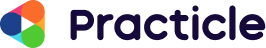## Learn the Guess and Check Method

What is the guess and check math strategy.

The Guess and Check Math strategy is one of the first few Math Heuristics that is introduced to Primary 3 students. Although some students may switch to more advanced techniques like the Assumption method in Primary 4, mastering this method is still going to be useful to those who don’t in their upper primary years. The main idea behind the Guess and Check method is to guess the answer to a problem and then check if the answer fits the given scenario. If it doesn’t, we’ll adjust our guess accordingly until all conditions are met.

Here’s what we’re going to cover:

• How does the Guess and check method work
• Examples of guess and check questions
• How to identify guess and check questions
• How to part 1: Building the guess and check table
• How to part 2: How to do the guess and check method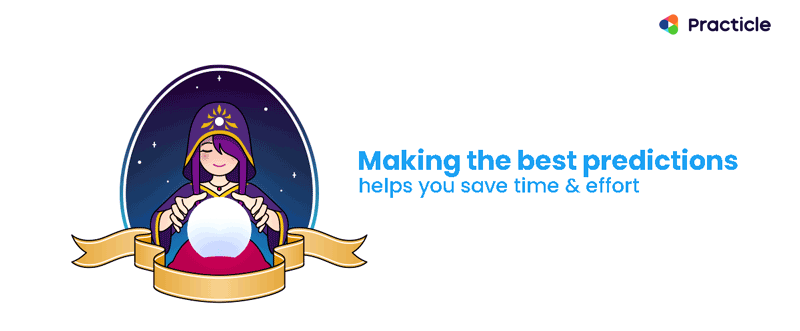## How does the Guess and Check Method work?

The Guess and Check problem solving strategy is a fairly easy way of solving problems. Think of it as a 3-step-approach:

## 1. Guess –> 2. Check –> 3. Repeat if needed

While we are guessing the numbers, we’ll need to learn how to make smart guesses. Knowing how to do that helps us minimize the number of guessing, making the process more efficient. We’ll see how to do that in a while.

## Examples of Guess and Check Questions

Here are some samples of how Guess and Check Math Questions can look like. Can you figure out what they have in common?

Primary 3 and 4 Math:

There are 15 cats and birds in a park. There are 42 legs altogether.

How many cats are there?

Primary 5 and 6 Math: Jay did 20 Math questions during his math practice. He received 5 marks for every correct answer and he got 2 marks deducted for every wrong answer. If Jay earned 35 experience points in total, how many questions did he answer wrongly?

## How do we identify questions that use the Guess and Check Method?

If you looked carefully, both problems that you see above has a total that’s made up of 2 kinds of items. on top of that, we also have some information about each item and we need to find the number of one of those items. Let’s use the lower primary math problem as an example and go through the Guess and Check method step by step.

## Part A: How to build the Guess and Check table the right way

Before we start guessing and checking, it’s always a good habit to build a guess and check table so that we know exactly what to look out for and keep things organised.

## Step 1: List the 2 items that is in the question

The first thing that we’ll want to do is to build our guess and check table is to think about what we want to solve for. This is the answer that we are going to guess and it goes into our first column. In our case, it’s going to be the number of cats. Next, we’ll list the number of the second item, which happens to be the number of birds as shown below: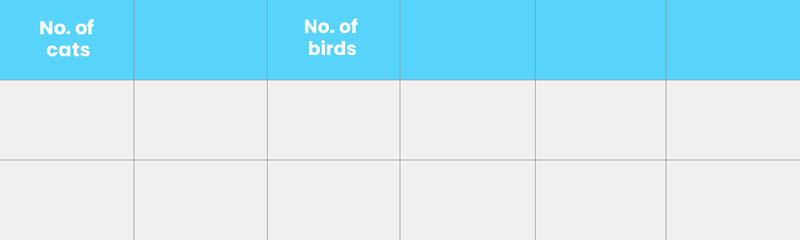## Step 2: List the common thing that the 2 items have

What do the cats and birds have in common? Legs. So the number of cats’ legs and the number of birds’ legs go into another 2 columns.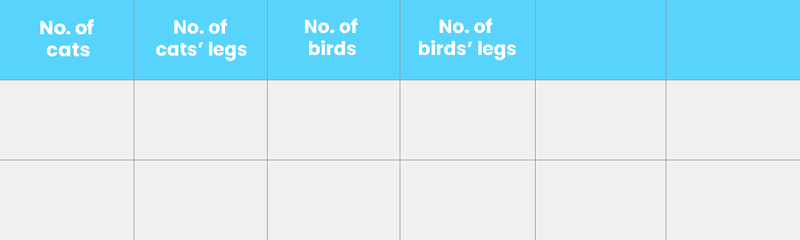## Step 3: Add in what we need to check

Time to think about the relationship between the columns and how they help us check if the answer that we have guessed is correct. For our answer to be right, the total number of legs that we have has to add up to 42 and the way that we calculate this is to add the number of cats’ legs to the number of birds’ legs. Let’s add another column to include the total number of legs and because we are going to use this to check of our guess is correct, we are going to add in another special column called “Check” to help us keep track of our progress.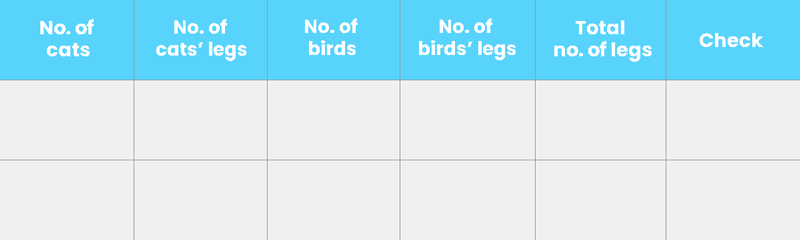## Is the Guess and Check Table really necessary?

When we are busy making guesses, it is easy to lose track of the numbers that we have tried along with their calculations. That’s where a guess and check table can really come in handy. Not only does a guess and check table help us organize our guesses in a neat visual way, it also prevents us from making careless mistakes.

## How to use the Guess and Check method efficiently

As you might have guessed, guessing and checking comes with a lot of hits and misses. Here’s the trick to keep our guesses to a minimal and make the guess and check method work better!

In our example, since we have 15 pets,  half of 15 would be approximately 7 or 8 (not 7.5 because we can’t have half a pet). Let’s pick 7 as our guess.

## Step 2: Filling up our guess and check table from left to right

Once we have our guess, it’s time to work through the columns of our guess and check table and decide if our guess is correct. If we had 7 cats and each puppy has 4 legs, we are going to have 7 x 4 cats’ legs = 28 cats’ legs. To find the number of birds, let’s subtract 7 from the total of 15 pets. This gives us 8 birds. 8 birds are going to have 8 x 2 birds’ legs, 16 legs in all. Let’s check the total number of legs we have against what is given in the problem. When we add 28 and 16, we are going to get a total of 44 legs. However, this is more than the 42 legs that we are given in the problem. Let’s make a note of that under the “Check” column.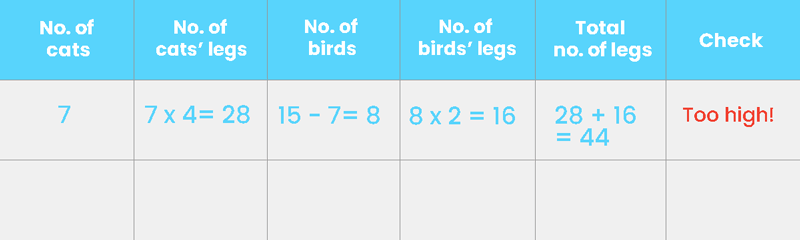## Step 3: Adjust our guess accordingly and check again

The next thing that we’ll want to do is to estimate how far our guess is to our target, and make a better guess. Comparing our guess which resulted in 44 legs against the 42 legs that we want, we can tell that our guess is very close. Since we want to reduce the number of legs, should we increase or decrease our second guess? Because the cats have more legs than birds and we want fewer legs, we’ll need to lower what we’ve guessed. When we work systematically across our guess and check table, this happens to be the answer that we are looking for!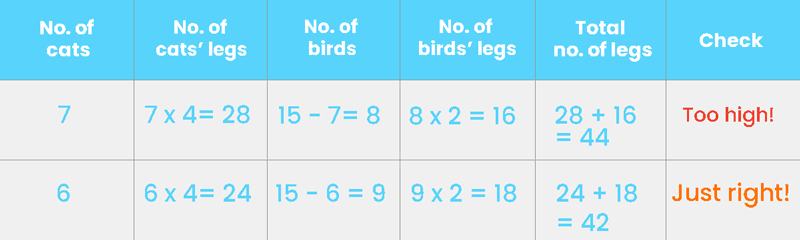All it takes for us to get the correct answer are 2 guesses, and this definitely didn’t happen by chance. When we made the first guess, it gave us some information about how far we are from the answer. This helped us make a better guess the second time. As you can see, we are making logical guesses instead of just guessing random numbers and trying our luck.

## And that’s how you do the Guess and Check method!

Using the Guess and Check method is good when you are dealing with smaller numbers which are easier to work with. However, it does take practice to improve the accuracy of your guesses and some children may take a longer time to do the calculations in between, giving room to possible careless mistakes. If you are looking for a faster alternative that involves fewer steps, you might want to check out the Assumption Method and see if that works better for you.

## Need more examples?

Check out how this Math video on how to use the guess and check method to solve a Primary 3 question.

Like what you see? Subscribe to our Youtube channel for more Practicle Singapore Math videos!Practicle is an online gamified Singapore Math adaptive learning platform that helps primary school children master Math through understanding and fun.

• EAF Certification
• Math heuristics guide
• Math problem sum guide

• A.I. Math Tutor
• Math Vision: Our video tutorials## Problem-Solving Strategies: Guess and Check, Work Backwards

More details.Looks like your browser is out-of-date.Internet Explorer• Kindergarten
• Greater Than Less Than
• Measurement
• Multiplication
• Place Value
• Subtraction
• Punctuation
• Cursive Writing

## Guess And Check

Guess And Check - Displaying top 8 worksheets found for this concept.

Some of the worksheets for this concept are Problem solving strategies guess and check work backward, 7 practice using guess and check, Unit 1 guess and check a decoding strategy, Group, Fractions section 1 iterating and partitioning, Polyas problem solving techniques, Polyas problem solving techniques, Reading comprehension practice test.

Found worksheet you are looking for? To download/print, click on pop-out icon or print icon to worksheet to print or download. Worksheet will open in a new window. You can & download or print using the browser document reader options.

## 1. Problem-Solving Strategies: Guess and Check, Work Backward

2. 7 practice using guess and check, 3. unit 1: guess and check: a decoding strategy, 5. fractions section 1: iterating and partitioning, 6. polyas problem solving techniques, 7. polyas problem solving techniques, 8. reading comprehension practice test.#### IMAGES

1. Solving Problems Using Guess and Check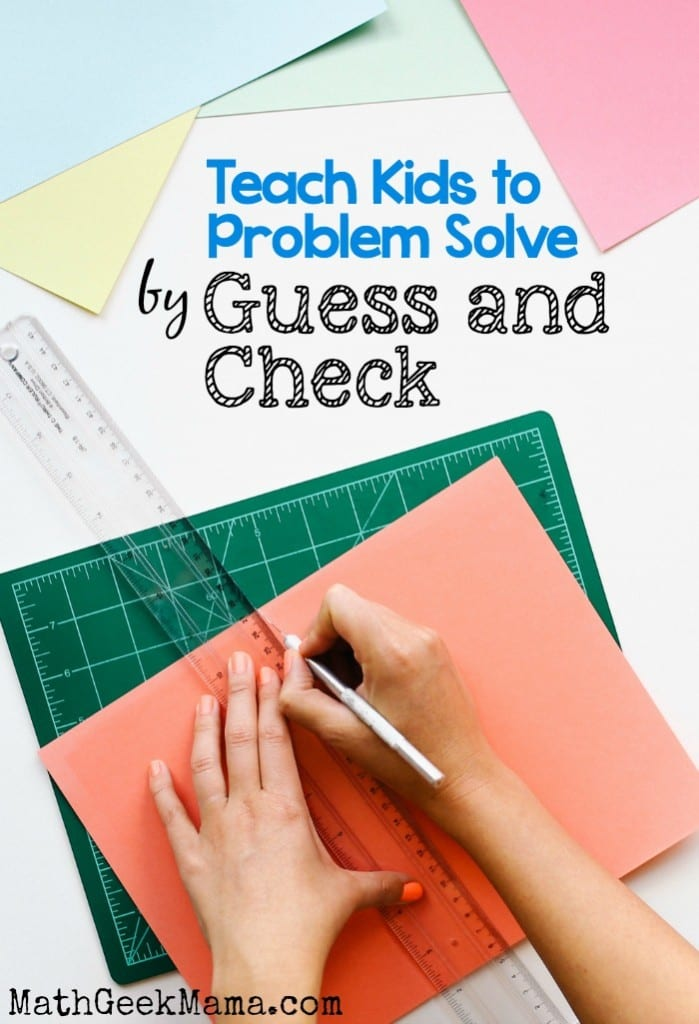2. Guess and check problem solving3. Guess, Check, and Revise to Solve Word Problems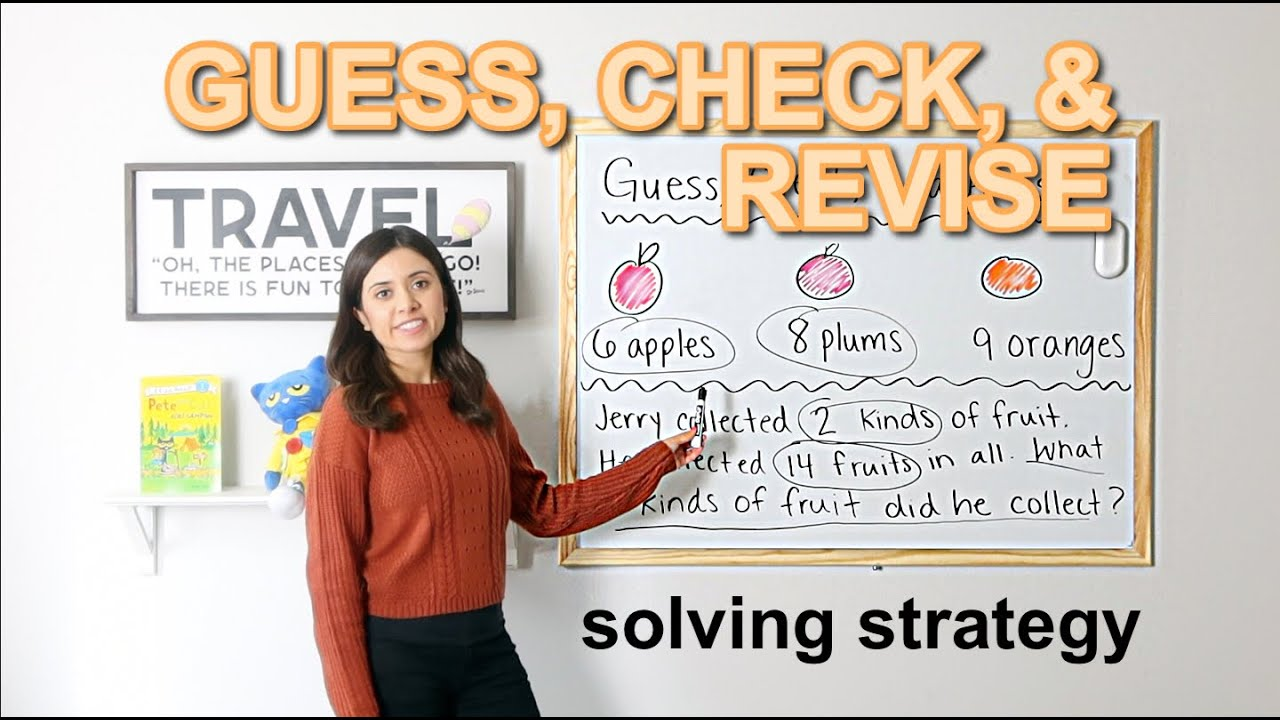4. Guess and check problem solving by Erin's Classroom Creations5. Problem Solving Strategy : Guess and Check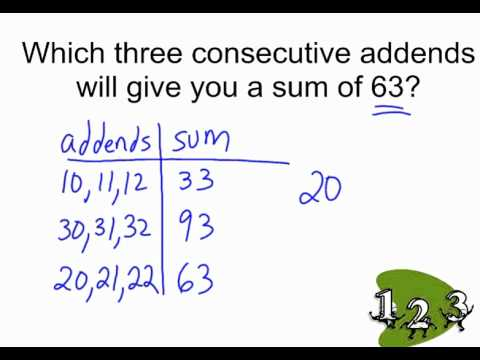6. How to Guess and Check Problems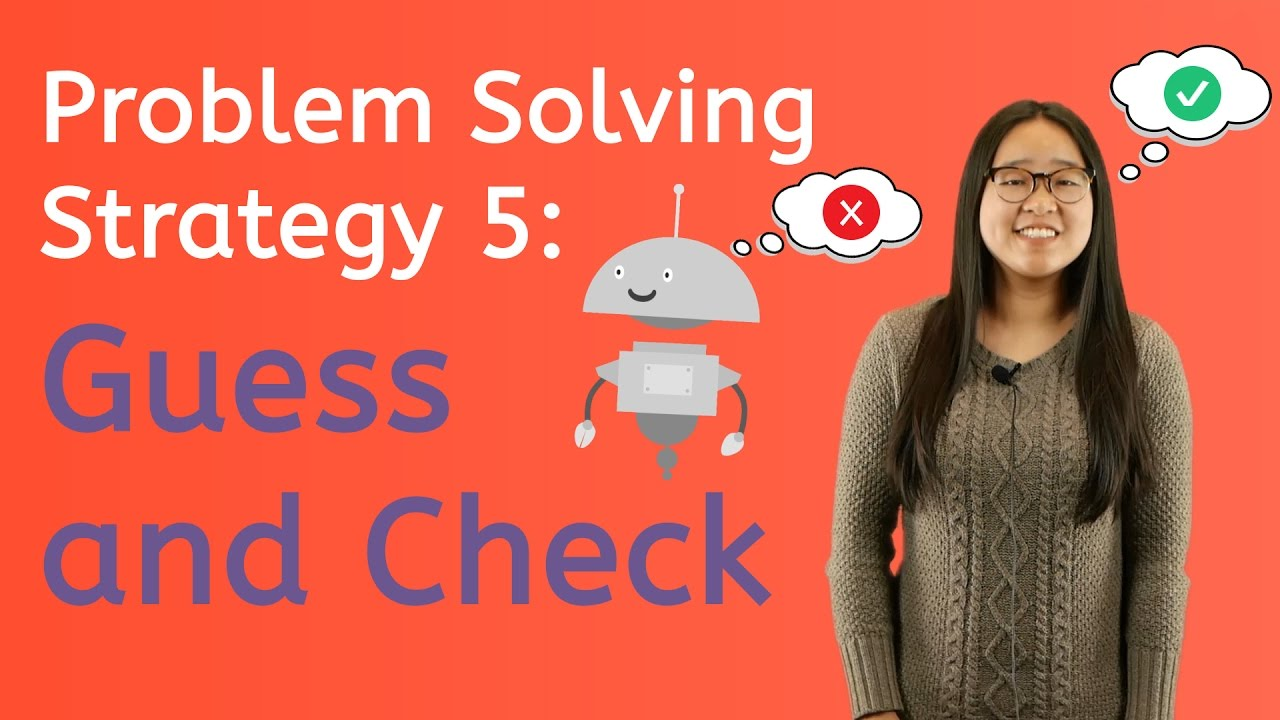#### VIDEO

1. Guess?? (check pin comnt 📌) #illusions #shorts #ytshorts

2. Can you Guess the Answer #shorts #quiz #Guess

3. improve problem solving in programming

4. Can you solve this test 👍 #shorts #test #maths

5. Problem Solving Skills

6. TRICKY Riddle

1. Problem Solving: Guess and Check

"Guess and Check" is a problem-solving strategy that students can use to solve mathematical problems by guessing the answer and then checking that the guess fits the conditions of the problem. For example, the following problem would be best solved using guess and check:

2. Solving Problems Using Guess and Check

Similarly, solving problems using guess and check is a process that requires logic and an understanding of the question so that it can be done in a way that is organized and time saving. So what does guess and check mean? To be more specific, this strategy should be called, "Guess, Check and Revise."

3. 2.5.3: Guess and Check, Work Backward

This lesson will expand your toolbox of problem-solving strategies to include guess and check and working backward. Let's begin by reviewing the four-step problem-solving plan: Step 1: Understand the problem. Step 2: Devise a plan - Translate. Step 3: Carry out the plan - Solve. Step 4: Look - Check and Interpret.

4. How Do You Solve a Problem Using the Guess, Check, and Revise Method?

Using logic is a strong approach to solving math problems! This tutorial goes through an example of using logical reasoning to find the answer to a word problem. Virtual Nerd's patent-pending tutorial system provides in-context information, hints, and links to supporting tutorials, synchronized with videos, each 3 to 7 minutes long.

5. Problem Solving Strategies

Guess and check is one of the simplest strategies. Anyone can guess an answer. If they can also check that the guess fits the conditions of the problem, then they have mastered guess and check. This is a strategy that would certainly work on the Farmyard problem described below but it could take a lot of time and a lot of computation.

6. Solving Problems With the Guess, Check & Revise Method

11K views The Steps Explained The first step in the guess, check, and revise method is to make an educated guess. Hmmm, what does it mean to make an educated guess? Notice, in our opening...

7. Problem Solving (Guess and Check)

This foundations of math video explains an example of the four-step process of problem solving using the method of guess and check. We look at understanding ...

8. Using the guess and check strategy for problem solving

Core Content In this lesson, we will begin to apply the guess and check strategy to a problem involving numbers within 15. Grid View List View Presentation Video Unsigned Video Signed Video Worksheet Transcript Numbers within 15: Counting up to 15 objects and putting them in order (Part 1)

9. Using the guess and check strategy for problem solving

Using the guess and check strategy for problem solving. In this lesson, we will begin to apply the guess and check strategy to a problem involving numbers within 15. Video. Play video. Unsigned Video Signed Video. Click on the play button to start the video. If your teacher asks you to pause the video and look at the worksheet you should:

10. Guess & Check Method

A good example of guess and check with plugging in numbers would be the example which was previously mentioned, 5 x - 1 = 34. If someone guesses the solution is 4, then: 5 x - 1 = 34. 5 (4) - 1 ...

11. Guess-Check-Improve Strategy: 2.5

Activity 1: Mystery number In this activity students use the guess-check-improve strategy to find unknown numbers. The importance of the improve phase of the guess-check-improve strategy is highlighted so that students can recognise that this strategy will provide answers more quickly than random guessing.

12. Guess and Check

Elementary Math Problem Solving - Guess and CheckIn this video, we explore one of eight problem-solving strategies for the primary math student. Students are...

13. Activities to Teach Students to Guess-And-Check Problems

Integrating real-life applications into guess-and-check problems can help students to understand how these problems can be used in everyday life. For example, students can solve problems related to budgeting and shopping, or calculating the number of ingredients needed for a recipe. 5. Problem-solving worksheets. Creating problem-solving ...

14. Quiz & Worksheet

Solving Problems With the Guess, Check & Revise Method - Quiz & Worksheet Lesson Quiz Course Try it risk-free for 30 days Instructions: Choose an answer and hit 'next'. You will receive your...

15. Using Guess, Check and Revise

Discipline: Math Domain: 4.MD Measurement and Data Grade(s): Grade 4 Cluster: Solve problems involving measurement and conversion of measurements from a larger unit to a smaller unit Standards: . 4.MD.1. Know relative sizes of measurement units within one system of units including km, m, cm, kg, g, lb, oz, l, ml, hr, min and sec.

16. Algebra Problem Solving: Guess & Check

Example #1 One of the times guess and check is especially useful is with quadratic equations. For example, this problem: Which of the following is a possible solution for the equation x2 - 28 =...

17. Guess and Check method, what is it?

The Guess and Check problem solving strategy is a fairly easy way of solving problems. Think of it as a 3-step-approach: 1. Guess -> 2. Check -> 3. Repeat if needed While we are guessing the numbers, we'll need to learn how to make smart guesses. Knowing how to do that helps us minimize the number of guessing, making the process more efficient.

18. IXL

08. hr. min. sec. SmartScore. out of 100. IXL's SmartScore is a dynamic measure of progress towards mastery, rather than a percentage grade. It tracks your skill level as you tackle progressively more difficult questions. Consistently answer questions correctly to reach excellence (90), or conquer the Challenge Zone to achieve mastery (100)!

19. problem solving

In the next attempt of distribution, 5040 cards were divided among x + 5 with each of the x grandchildren having 75 cards less from the first attempt of distribution. So, 5040 x + 5 = y − 75 ... (II) Solve equation (I) and (II) to get the answer. To guess and check, start with an initial estimate, say 10 total grandchildren.

20. Problem-Solving Strategies: Guess and Check, Work Backwards

Problem-Solving Strategies: Guess and Check, Work Backwards - FUSE - Department of Education & Training FUSE is being decommissioned and will transition to read-only mode from 31 July and will be replaced by Arc Learning in Term 4 2023. Learn more about: FUSE TRANSITION ARC LEARNING Mathematics ID: 44HJDB

21. Algebra Problem Solving: Guess & Check

Instructions: Choose an answer and hit 'next'. You will receive your score and answers at the end. question 1 of 3. Which of the following is a possible solution for the equation x2 + x = 72? Use ...

22. Guess and Check problem solving: Teach, Together, Try

guess and check

23. Guess And Check Worksheets

Guess And Check. Guess And Check - Displaying top 8 worksheets found for this concept. Some of the worksheets for this concept are Problem solving strategies guess and check work backward, 7 practice using guess and check, Unit 1 guess and check a decoding strategy, Group, Fractions section 1 iterating and partitioning, Polyas problem solving ...